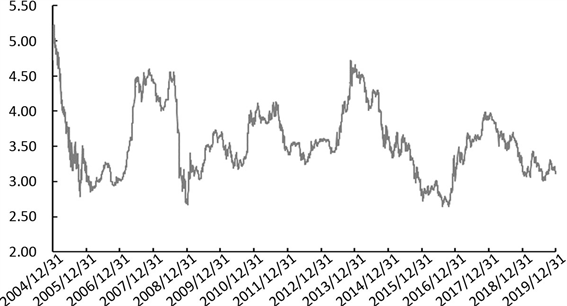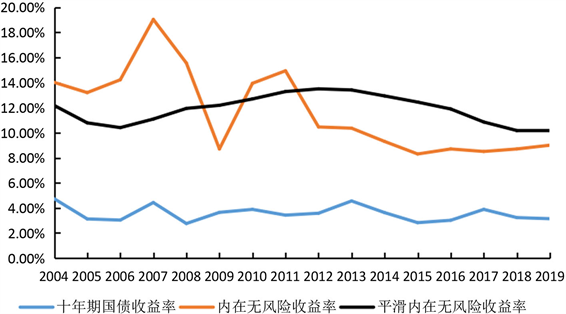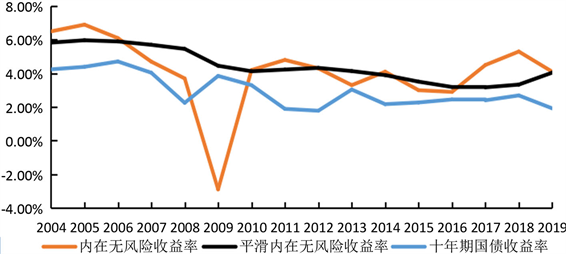﻿ 中国蓝筹股估值中的股息永续增长率

# 中国蓝筹股估值中的股息永续增长率On the Perpetual Growth Rate of Dividends for Chinese Blue-Chip Stocks

Abstract: The investment return of blue-chip stocks is of great significance to investors’ decision-making. Due to the importance of the perpetual dividend growth rate when we estimate the expected return of stocks, using the data of the CSI 300 Index, this paper discusses, with the benchmarking of the his-torical dividend growth rate, the referencing of the risk-free rate, of the intrinsic risk-free rate, of the smoothed intrinsic risk-free rate, and of the potential return growth rate (put the compound annual growth rate of CPI and GDP together), as the parameter of the perpetual growth rate of divi-dends. This author argues that, the intrinsic risk-free rates and the potential return growth rate could be better parameters of the perpetual growth rate of dividends in China.

1. 引言

2. 模型与比较基准

2.1. 三阶段股利贴现模型

$V=\underset{t=1}{\overset{\infty }{\sum }}\frac{{D}_{t}}{{\left(1+r\right)}^{t}}=\frac{{D}_{1}}{1+r}+\frac{{D}_{2}}{{\left(1+r\right)}^{2}}+\cdots +\frac{{D}_{m}}{{\left(1+r\right)}^{m}}+\cdots +\frac{{D}_{n}}{{\left(1+r\right)}^{n}}+\frac{{D}_{n}\ast \left(1+g\right)}{\left(r-g\right)\ast {\left(1+r\right)}^{n}}$

V：研究期(2004~2019)内每年度最后一个交易日沪深300的总市值。

Dt：第t年的股东回报。股东回报 = 现金分红 + 股票回购。

g：股息永续增长率。在该模型的第三阶段中，假设第n年之后，每年的股东回报以固定的增长率g永续增长。

r：折现率，即蓝筹股(沪深300)的预期股权投资回报。

Table 1. Return to shareholders and net profit of CSI 300: 2004-2021

2.2. 参数的选取

2.3. 比较基准：历史股息增长率Table 2. Historical dividend growth rates for CSI 300: 2004-2018

2.4. 预期股权回报(据历史股息增长率)

Table 3. Historical dividend growth rate and related expected return of CSI 300: 2004-2019

3. 参数选择

3.1. 无风险收益率Figure 1. 10-year treasury yield of China: Dec. 31, 2004-Dec. 31, 2019 (%)Table 4. Risk-free rate and related expected return of CSI 300: 2004-2019

3.2. 内在无风险收益率

Table 5. Intrinsic risk-free rate and related expected return of CSI300: 2004-2019

3.3. 平滑内在无风险收益率

Table 6. Smoothed intrinsic risk-free rate of China: 2004-2019Figure 2. Parameters of Chinese perpetual growth rate of dividends 2004-2019Table 7. Smoothed intrinsic risk-free rate and related expected return of CSI 300: 2004-2019

3.4. 回报潜在增长率

$\text{CAGR}=\left(现有价值/基期价值\right)^\left(\text{1}/年数\right)-1$

Table 8. Potential return growth rate of China blue-chip stocks: 2004-2019Table 9. Potential return growth rate and related expected return of CSI 300: 2004-2019

4. 比较与讨论Table 10. Parameters of perpetual growth rate of dividends and related expected return of CSI 300: 2004-2019

Table 11. Parameters of American perpetual growth rate of dividends: 2004-2019Figure 3. Parameters of the United States perpetual growth rate of dividends: 2004-2019

NOTES

1引自“Academic studies assume that dividend growth for the overall market cannot exceed the total economy's growth over the long term. If the economy—as measured by gross domestic product (GDP) or national income—grows at 4%, then studies assume that markets cannot collectively outpace this growth rate. So, if you start with an assumption that the market's current valuation is approximately correct and you set the economy’s growth as a limit on long-term dividend growth (or earnings or earnings per share growth), a real equity premium of 4% or 5% is pretty much impossible to exceed.”

2公式：收益率标准差*(250^0.5)。

 楚义芳, 许源, 夏思博. 中国蓝筹股的长期股权投资回报[J]. 金融, 2019, 9(6): 611-624.

 Harper, D.R. (2019) Calculating the Equity Risk Premium. https://www.investopedia.com/investing/calculating-equity-risk-premium/

 Harper, D.R. (2020) The Equity Risk Premium: More Risk for Higher Returns. https://www.investopedia.com/articles/04/012104.asp

 Segal, T. (2019) Build a Dividend Portfolio That Grows with You. https://www.investopedia.com/articles/stocks/07/build_dividend_portfolio.asp

 Harper, D.R. (2019) Build a Divi-dend Portfolio That Grows with You. https://www.investopedia.com/articles/stocks/07/build_dividend_portfolio.asp

 Damodaran, A. (2018) Data Update 2: The Buoyancy of US Equities. https://seekingalpha.com/article/4136522-january-2018-data-update-2-buoyancy-of-u-s-equities

 Damodaran, A. (2018) Testing Times: Market Turmoil and Investment Serenity. https://seekingalpha.com/article/4145458-testing-times-market-turmoil-and-investment-serenity

 Damodaran, A. (2019) Data Update 1: A Reminder That Equities Are Risky, In Case You Forgot. https://seekingalpha.com/article/4231190-january-2019-data-update-1-reminder-equities-are-risky-in-case-you-forgot

 Damodaran, A. (2019) Data Update 2: The Message from Bond Markets. https://seekingalpha.com/article/4231974-january-2019-data-update-2-message-from-bond-markets

Top